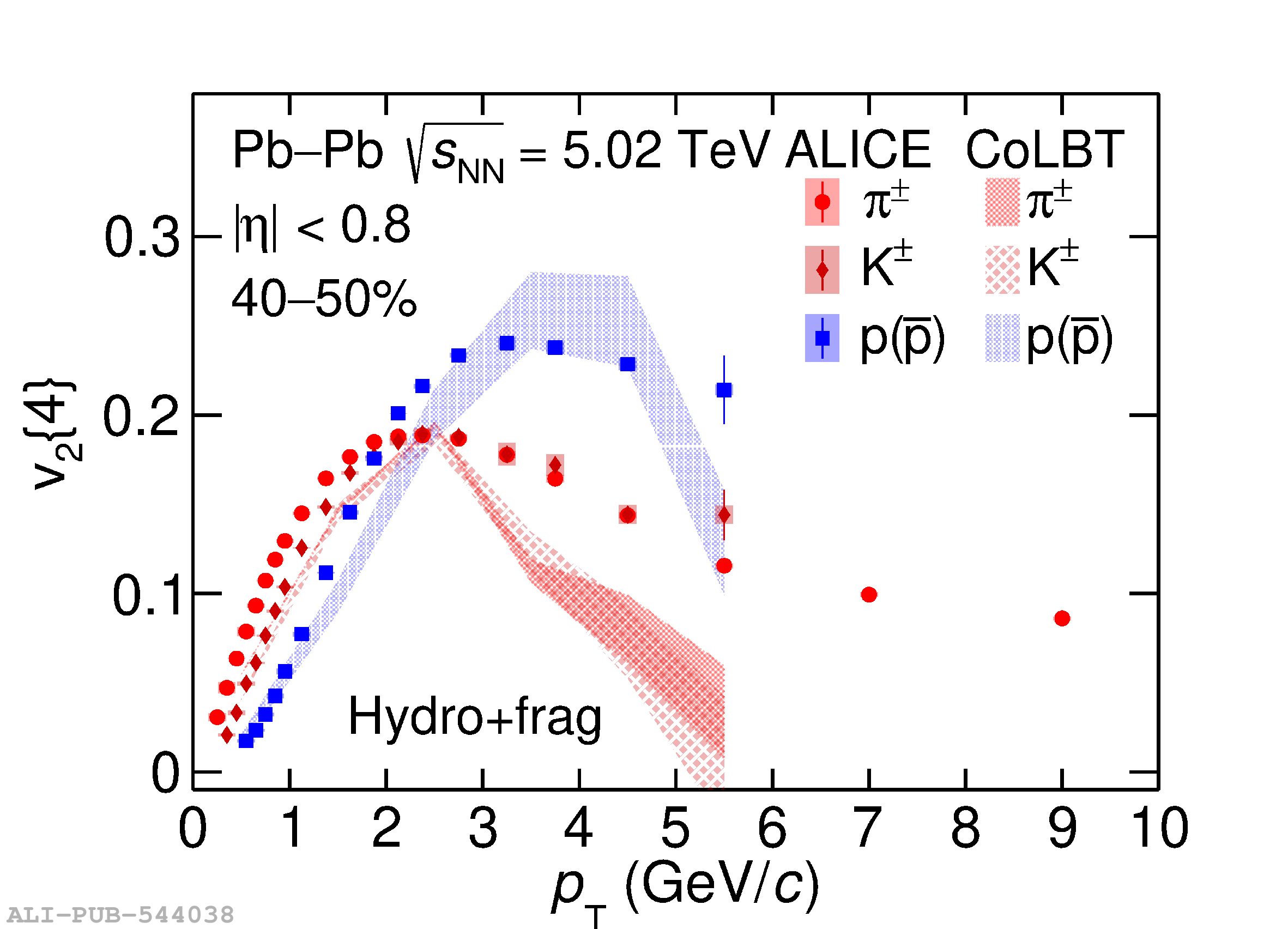# Figure 10

 The \pt-differential $v_2\{4\}$ for \pipm{}, \kapm{}, and p+\pbar{} measured in Pb--Pb collisions at $\sqrt{s_{\mathrm{NN}}} = 5.02$ TeV compared with expectations of the same quantity from the CoLBT hydrodynamic model without quark coalescence  in 40--50\% centrality interval. The vertical error bars and the filled boxes represent statistical and systematic uncertainties of the data, respectively. The thickness of the model curves reflect the uncertainties of the hydrodynamic calculations.